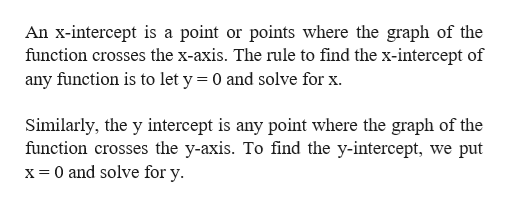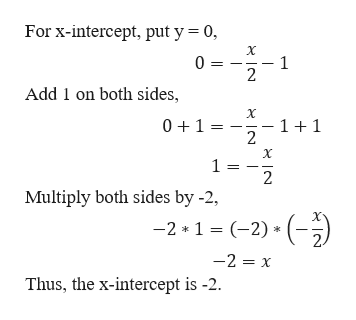Question
12 views
check_circle

Step 1

Given an equation

Step 2

Note:help_outlineImage TranscriptioncloseAn x-intercept is a point or points where the graph of the function crosses the x-axis. The rule to find the x-intercept of any function is to let y = 0 and solve for x. Similarly, the y intercept is any point where the graph of the function crosses the y-axis. To find the y-intercept, we put x= 0 and solve for y. fullscreen
Step 3

Now, using the defini...help_outlineImage TranscriptioncloseFor x-intercept, put y = 0, X 1 0 = 2 Add 1 on both sides, 01 11 2 1 = 2 Multiply both sides by -2 -2 1 (-2)) -2 x Thus, the x-intercept is -2 fullscreen

### Want to see the full answer?

See Solution

#### Want to see this answer and more?

Solutions are written by subject experts who are available 24/7. Questions are typically answered within 1 hour.*

See Solution
*Response times may vary by subject and question.
Tagged in
MathAlgebra

### Other